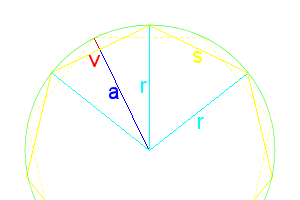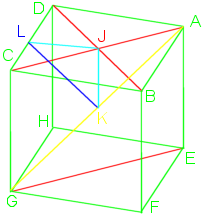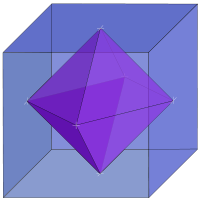# Prisms and Antiprisms

In geometry, a prism is a polyhedron with an n-sided polygonal base, another congruent parallel base (with the same rotational orientation), and n other faces (necessarily all parallelograms) joining corresponding sides of the two bases. Prisms are named for their base, so a prism with a pentagonal base is called a pentagonal prism.

In regular prisms, all side faces (all squares) are at right angles to the bases. The number of prisms is infinite, approaching a circular base.

The interior angles of the base faces is $$\frac{180(n-2)}{n}$$, where n is the number of sides. This is really the only “difficult” math property of prisms.

Some prisms are not normally considered or called prisms, such as a square prism is just a cube.

If instead of connecting the bases with squares, you connect them with equilateral triangles, you then get antiprisms.

For n=2, the digonal antiprism is a degenerate case, as there is no base polygon, only a line segment. Otherwise the top face is a duplicate of the bottom, only rotated 180°/n.Figure 1: Polygon Dimensions

For dihedral angles for antiprisms, things are a lot more complicated than prisms.

A regular polygon always has the following properties:

\begin{align} radius\ r&=DB=\frac{s}{2\sin\frac{180^\circ}{n}} = \frac{a}{\cos\frac{180^\circ}{n}} \\ apothem\ a &=\overline{DE}=r\cdot\cos\frac{180^\circ}{n}=\frac{s}{2\tan\frac{180^\circ}{n}} \\ side\ s&=r\cdot2\sin\frac{180^\circ}{n}=a\cdot 2\tan\frac{180^\circ}{n}=AB=BC=FG \\ angle\ \theta &=\frac{360^\circ}{n} \\sagitta\ v&=r-a=r(1-\cos\frac{360}{2n})=r(2\sin^2\frac{360}{4n})=\frac{s(1-\cos\frac{360}{2n})}{2\sin\frac{360}{2n}}=\frac{s(\sin^2\frac{360}{4n})}{\sin\frac{360}{2n}}=s\frac12\tan\frac{90}{n}=\overline{BE} \end{align}

The apothem is the distance from the center to the midpoint of any side. The difference between the radius and apothem is called the sagitta, also sometimes called the versine function ($$versin\ \mu=1-\cos\mu=2\sin^2\frac12\mu)$$. The antiprism’s sides will be deflected away from 90° by the length of BE (or v). The dotted line is the location of the second base, always rotated θ/2 to that of the first base.

Normally it would be odd trying to calculate the radius of a circle, but we are defining our polygon based on its side length being one unit.

If we draw a line at the midpoint of each triangle all the way around, we get a regular polygon with 2n sides, parallel to each base.

The height of the triangle is line BE2, $$\frac{\sqrt3}{2}$$. We do not yet know the height of the antiprism ($$\overline{E_1E_2}$$), but can find it.

\begin{align} (\overline{BE_1})^2+(\overline{E_1 E_2})^2 &=(\overline{BE_2})^2 \\ (\overline{E_1 E_2})^2 &=(\overline{BE_2})^2-(\overline{BE_1})^2 \\ &=\left(\frac{\sqrt3}{2}\right)^2-\left(s\frac12\tan\frac{90}{n}\right)^2 \\ &=\frac34-s^2\cdot\frac14\tan^2\frac{90}{n} \\ &=\frac{3-s^2\tan^2\frac{90}{n}}{4} \\ \overline{E_1 E_2} &=\frac12\sqrt{3-s^2\tan^2\frac{90}{n} } \end{align}

This is as simplified as we can get without deciding on how many sides the base will have. The height of an antiprism must always be less than $$\frac12 s\sqrt3$$, the height of a regular triangle.

Here are a few of the first heights for n:

 n θ Height 2 45 $$\frac1{\sqrt2}$$ 3 60 $$\sqrt{\frac23}$$ 4 90 $$\sqrt{\frac12}$$ 5 36 $$\sqrt{\frac{5+\sqrt5}{10}}$$ 6 30 $$\sqrt{\sqrt3-1}$$ 8 22.5 $$\sqrt{\sqrt{5+\frac{7\sqrt2}{2}} -1-\sqrt2}$$ 10 18 $$\frac12\sqrt{-8-4\sqrt5-2(1+\sqrt5)\sqrt{5+2\sqrt5}}$$

For the dihedral angles, ∠E1BE2 is the same as ∠Ψ, and $$\cos\angle BE_2D_2 =-\cos\angle\Psi$$.

\begin{align} \cos\angle E_1BE_2 &=\frac{(BE_1)^2+(BE_2)^2-(E_1 E_2)^2}{2[(BE_1)(BE_2)]} \\ &=\frac{\left(\frac12\tan\frac{90}{n}\right)^2+\left(\frac{\sqrt3}{2}\right)^2-\left(\frac12\sqrt{3-\tan^2\frac{90}{n} }\right)^2}{2\cdot\frac12\tan\frac{90}{n}\cdot\frac{\sqrt3}{2}} \\ &=\frac{ \frac{\tan^2\frac{90}{n}}{4}+\frac34-\frac{3-\tan^2\frac{90}{n} }{4} }{\frac{\sqrt3}{2}\cdot\tan\frac{90}{n}} \\ &=\frac{ \frac{2\tan^2\frac{90}{n}}{4} }{\frac{\sqrt3}{2}\cdot\tan\frac{90}{n}}=\frac{ \frac12\tan^2\frac{90}{n} }{\frac{\sqrt3}{2}\cdot\tan\frac{90}{n}} \\ &= \frac{\tan^2\frac{90}{n}}{2} \cdot \frac{2}{\sqrt3\cdot\tan\frac{90}{n}} \\ &= \frac{\tan^2\frac{90}{n}}{\sqrt3\cdot\tan\frac{90}{n}} \\ &= \frac{\tan\frac{90}{n}}{\sqrt3} \\ &= \frac1{\sqrt3}\cdot\tan\frac{90}{n} \\ \cos\angle BE_2D_2&=-\frac1{\sqrt3}\cdot\tan\frac{90}{n} \end{align}

Here are the first few dihedrals for n:

 n $$\angle E_1BE_2$$ $$\angle BE_2D_2$$ 2 54.735° 125.264° 3 70.528° 109.471° 4 76.163° 103.836° 5 79.187° 100.812° 6 81.101° 98.8994° 8 83.405° 96.5945°

For the side-to-side dihedrals, let’s draw a line from point C, straight down, equal to the height, and call this point K. K is in the same plane as the bottom base. Looking at Figure 1, we can see that ∠KDF is $$\frac32\theta$$, or $$\frac{540}{n}$$. Connecting F and K, we get a line of $$2r\sin\frac{540}{2n}= s\cdot\frac{sin\frac{270}{n}}{\sin\frac{180}{n}}$$. Note $$\overline{FK}$$ is coplanar with G and E2, but does not go through either point.

We can now find the distance between C and F,

\begin{align} (CF)^2&=(CK)^2+(FK)^2 \\ &=\frac{3-s^2\tan^2\frac{90}{n}}{4} + s^2\cdot\frac{\sin^2\frac{270}{n}}{\sin^2\frac{180}{n}} \\ (CF) &=\sqrt{\frac{3-s^2\tan^2\frac{90}{n}}{4} + s^2\cdot\frac{\sin^2\frac{270}{n}}{\sin^2\frac{180}{n}} } \end{align}

In Figure 2, we see that $$CL=LF=BE_2=\frac{\sqrt3}{2}$$. We can now find ∠CLF, the dihedral, with s=1 factored out,

\begin{align} \cos\angle CLF &=\frac{(CL)^2+(LF)^2- (CF)^2}{2\cdot CL\cdot LF} \\ &=\frac{(\frac{\sqrt3}{2})^2+(\frac{\sqrt3}{2})^2-(CF)^2 }{2\cdot (\frac{\sqrt3}{2})(\frac{\sqrt3}{2})} \\ &=\frac{\frac34+\frac34-(CF)^2 }{\frac32} \\ &=\left[\frac64-(CF)^2\right]\div\frac32=\left[\frac32-(CF)^2\right]\cdot\frac23 \\ &=1-\frac23(CF)^2 \\ &=1-\frac23\left(\frac{3-\tan^2\frac{90}{n}}{4} + \frac{\sin^2\frac{270}{n}}{\sin^2\frac{180}{n}} \right) \end{align}

Multiplying out the CF2 is really messy, best to reduce the other parts as much as possible, then square.

Here are the first few dihedral angles for n:

 n $$\cos\angle CLF$$ ∠CLF 2 $$\frac13$$ 70.528779 3 $$-\frac13$$ 109.47122 4 $$\frac{1-2\sqrt2}{3}$$ 127.552 5 $$-\frac{\sqrt5}{3}$$ 138.189685

# The Cube

The cube is probably the most recognized and best known of and 3D shape. Kids young enough not to even know how to talk will still know about cubes, they play with multicolored wooden blocks. Older kids may tackle the Rubik’s Cube puzzle or play Yahtzee with dice. Then they may graduate to working in a cubicle as adults.

We use the cube as a representation of the space around us. Pepsi was briefly sold in 24-can “cubes.” Apple’s G4 computer was cubic.

Al Kaaba (literal English, the cube) is a cuboid shaped building in Mecca and the most sacred site to Islam.

Salt grows into cubic crystals.

Stones chipped and shaped into cubes have been found that date back 100’s of thousands of years.

Star Trek’s fictional, I hope, Borg traveled through space in a cube shaped ship.

There are several prisons with the nickname “the cube,” despite not having walls as tall as they are wide.

They are literally, all around us. We have have such a familiarity with the cube that we don’t even have to think about its qualities or structure. We just know it.

Therefore, having a page devoted to the cube may seem like a waste of space, but I’m certain that there is still knowledge we can gain from re-examining the world around us.The cube is a 3 dimensional solid object made of 6 squares (variously called faces, facets, or sides), each meeting at right (90°) angles with two others. It is one of the 5 Platonic solids and can also be referred to as a regular hexahedron or a square prism.

Like a sphere is the set of all points equidistant from the origin, the cube is the set of points that make up the square faces, it has no volume, although we usually refer to the volume it contains.

If we call the edge length S, then  each face will have area $$S^2$$, so the cube will have surface area $$6*S^2$$. The internal volume is $$S^3$$.

Many different shapes can tile 2D space, squares, hexagons, various triangles. The cube is the only object that can regularly tile Euclidean 3D space.

Using formulas already mentioned, vertex edge angles are: $$\Large\cos\mu=\frac{cos90}{cos45}=\frac{0}{\frac{1}{\sqrt2}}=0, \mu=90^\circ$$.

Using a cube with vertexes named A-H, as in the image, with edge length of 1, we can then calculate other lengths, easily done with right triangles and the Pythagorean theorem. Actually, it is even easier as almost all will be 45° right triangles.

Drawing a diagonal across a face from B to D, we get a right triangle with BD length $$\sqrt2$$. Drawing diagonal AC crosses at the midpoint J.

Drawing a spacial diagonal through the cube from A to G, triangle AEG lengths would be $$1,\sqrt2, and \sqrt3$$. Midpoint of AG is K which is also the spacial midpoint of the cube. AK and GK are $$\frac{\sqrt3}{2}$$, the radius of a sphere surrounding the cube.To find JK:
\begin{align} (AJ)^2+(JK)^2&=(AK)^2\\ (JK)^2&=(AK)^2-(AJ)^2\\ &=\left(\frac{\sqrt3}{2}\right)^2-\left(\frac{\sqrt2}{2}\right)^2\\ &=\frac34-\frac24\\ &=\frac14\\ JK&=\frac12\end{align} so JK=$$\frac12$$. This is the radius of an inscribed sphere.

The midpoint of CD is L. Line JL would 1/2 the edge length, equal to JK, so $$KL=\frac{\sqrt2}{2}=\frac1{\sqrt2}$$. Angle JLK is 45°, one half the dihedral (face to face) angle.

If you join the mid-face points (all the J’s), you would then get the cube’s dual shape called the octahedron. Similarly, joining the midpoints of the octahedron’s face would give you a cube. An octahedron inside a cube of edge length S would have edges of length $$S\cdot\frac1{\sqrt2}$$ and an octahedron outside the cube would have edge length of $$S\cdot\sqrt2$$. The octahedra would have volumes of $$S^3\cdot\frac13$$ and $$S^3\cdot\frac23$$, respective.